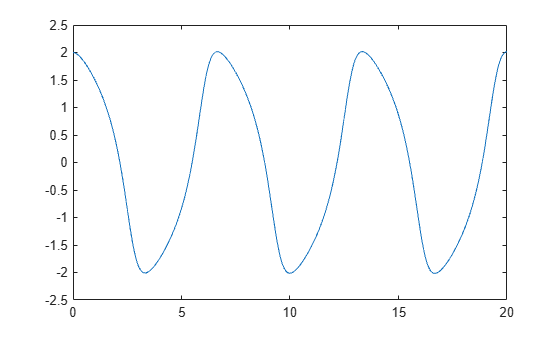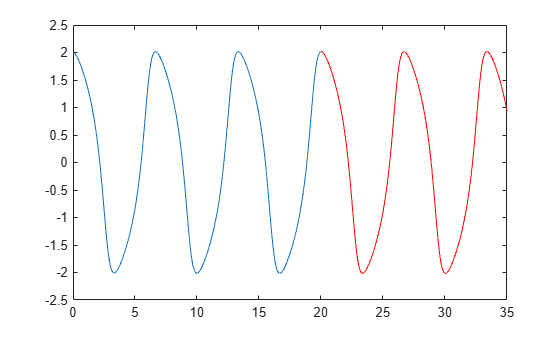# odextend

Extend solution to ODE

## Syntax

``solext = odextend(sol,odefun,tfinal)``
``solext = odextend(sol,[],tfinal)``
``solext = odextend(sol,odefun,tfinal,y0)``
``solext = odextend(sol,odefun,tfinal,y0,options)``

## Description

example

````solext = odextend(sol,odefun,tfinal)` extends the solution `sol` by integrating `odefun` from `sol.x(end)` to `tfinal`, using the same ODE solver that created `sol`. The function `odefun` can be different than the original function used to compute `sol`. The lower bound for the independent variable in `solext` is the same as in `sol`, that is, `sol.x(1)`. By default, `odextend` uses:The initial conditions `y = sol.y(:,end)` for the subsequent integration.The same integration properties and additional input arguments that the ODE solver originally used to compute `sol`. This information is stored in the solution structure `sol` and is later returned in `solext`. Unless you want to change these values, you do not need to pass them to `odextend`.```
````solext = odextend(sol,[],tfinal)` extends the solution to the same ODE function that was solved to obtain `sol`.```
````solext = odextend(sol,odefun,tfinal,y0)` specifies new initial conditions `y0` for the extended integration instead of using `sol.y(:,end)`.For the `ode15i` solver: `y0` must be an m-by-2 matrix containing column vectors of initial conditions for the solution components and their derivatives, ```y0 = [yinit ypinit]```.```
````solext = odextend(sol,odefun,tfinal,y0,options)` uses the integration options defined by `options`, which is an argument created using the `odeset` function. The specified options override the options that the ODE solver originally used to compute `sol`. You can optionally specify ```y0 = []``` to use default initial conditions.```

## Examples

collapse all

The van der Pol equation is a second order ODE

`${y}_{1}^{\prime \prime }-\mu \left(1-{y}_{1}^{2}\right){y}_{1}^{\prime }+{y}_{1}=0.$`

Solve the van der Pol equation with $\mu =1$ using `ode45`. The function `vdp1.m` ships with MATLAB® and encodes the equations. Specify a single output to return a structure containing information about the solution, such as the solver and evaluation points.

```tspan = [0 20]; y0 = [2 0]; sol = ode45(@vdp1,tspan,y0)```
```sol = struct with fields: solver: 'ode45' extdata: [1x1 struct] x: [1x60 double] y: [2x60 double] stats: [1x1 struct] idata: [1x1 struct] ```

Use `linspace` to generate 250 points in the interval `[0 20]`. Evaluate the solution at these points using `deval`.

```x = linspace(0,20,250); y = deval(sol,x);```

Plot the first component of the solution.

`plot(x,y(1,:))`Extend the solution to ${t}_{f}=35$ using `odextend` and add the result to the original plot.

```sol_new = odextend(sol,@vdp1,35); x = linspace(20,35,350); y = deval(sol_new,x); hold on plot(x,y(1,:),'r')```## Input Arguments

collapse all

Solution structure, specified as a structure returned by an ODE solver. When you call an ODE solver with a single output argument, it returns a solution structure.

Example: `sol = ode45(myode,tspan,y0)`

Data Types: `struct`

Function to solve, specified as a function handle. Use this input to extend the solution using a new or modified ODE function. To continue using the original ODE function used to create the solution structure `sol`, specify `odefun` as an empty input `[]`.

Data Types: `function_handle`

Final integration time, specified as a scalar.

Data Types: `single` | `double`

Initial conditions, specified as a scalar, vector, or matrix. By default `odextend` uses the initial conditions ```y = sol.y(:,end)``` to extend the integration. Use this input to specify new initial conditions for the extended integration.

For the `ode15i` solver: `y0` must be an m-by-2 matrix containing column vectors of initial conditions for the solution components and their derivatives, ```y0 = [yinit ypinit]```.

Data Types: `single` | `double`

Options structure. By default, `odextend` uses the same options and additional inputs as the ODE solver originally used to compute `sol`. Use this input to specify a new options structure that overrides the options used to create `sol`.

Use the `odeset` function to create or modify an ODE options structure.

Data Types: `struct`

## Output Arguments

collapse all

Extended solution, returned as a structure. Use this structure with the `deval` function to evaluate the solution at any point in the interval `[t0 tf]`. The `solext` structure array always includes these fields:

Structure FieldDescription

`sol.x`

Row vector of the steps chosen by the solver.

`sol.y`

Each column `sol.y(:,i)` contains the solution at time `sol.x(i)`.

`sol.solver`

Solver name.

Additionally, if you specify the `Events` option and events are detected, then `solext` also includes these fields:

Structure FieldDescription

`sol.xe`

Points when events occurred. `sol.xe(end)` contains the exact point of a terminal event, if any.

`sol.ye`

Solutions that correspond to events in `sol.xe`.

`sol.ie`

Indices into the vector returned by the function specified in the `Events` option. The values indicate which event the solver detected.